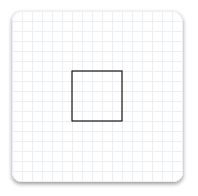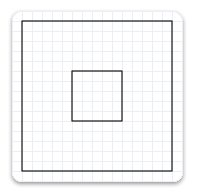# ID2D1Factory::CreateTransformedGeometry(ID2D1Geometry*,constD2D1_MATRIX_3X2_F*,ID2D1TransformedGeometry**) method (d2d1.h)

Transforms the specified geometry and stores the result as an ID2D1TransformedGeometry object.

## Syntax

``````HRESULT CreateTransformedGeometry(
ID2D1Geometry            *sourceGeometry,
const D2D1_MATRIX_3X2_F  *transform,
ID2D1TransformedGeometry **transformedGeometry
);
``````

## Parameters

`sourceGeometry`

Type: [in] ID2D1Geometry*

The geometry to transform.

`transform`

Type: [in] const D2D1_MATRIX_3X2_F*

The transformation to apply.

`transformedGeometry`

Type: [out] ID2D1TransformedGeometry**

When this method returns, contains the address of the pointer to the new transformed geometry object. The transformed geometry stores the result of transforming sourceGeometry by transform.

## Return value

Type: HRESULT

If this method succeeds, it returns S_OK. Otherwise, it returns an HRESULT error code.

## Remarks

Like other resources, a transformed geometry inherits the resource space and threading policy of the factory that created it. This object is immutable.

When stroking a transformed geometry with the DrawGeometry method, the stroke width is not affected by the transform applied to the geometry. The stroke width is only affected by the world transform.

## Examples

The following example creates an ID2D1RectangleGeometry, then draws it without transforming it. It produces the output shown in the following illustration.``````hr = m_pD2DFactory->CreateRectangleGeometry(
D2D1::RectF(150.f, 150.f, 200.f, 200.f),
&m_pRectangleGeometry
);
``````

The next example uses the render target to scale the geometry by a factor of 3, then draws it. The following illustration shows the result of drawing the rectangle without the transform and with the transform; notices that the stroke is thicker after the transform, even though the stroke thickness is 1.``````// Transform the render target, then draw the rectangle geometry again.
m_pRenderTarget->SetTransform(
D2D1::Matrix3x2F::Scale(
D2D1::SizeF(3.f, 3.f),
D2D1::Point2F(175.f, 175.f))
);

m_pRenderTarget->DrawGeometry(m_pRectangleGeometry, m_pBlackBrush, 1);
``````

The next example uses the CreateTransformedGeometry method to scale the geometry by a factor of 3, then draws it. It produces the output shown in the following illustration. Notice that, although the rectangle is larger, its stroke hasn't increased.`````` // Create a geometry that is a scaled version
// of m_pRectangleGeometry.
// The new geometry is scaled by a factory of 3
// from the center of the geometry, (35, 35).

hr = m_pD2DFactory->CreateTransformedGeometry(
m_pRectangleGeometry,
D2D1::Matrix3x2F::Scale(
D2D1::SizeF(3.f, 3.f),
D2D1::Point2F(175.f, 175.f)),
&m_pTransformedGeometry
);
``````
``````// Replace the previous render target transform.
m_pRenderTarget->SetTransform(D2D1::Matrix3x2F::Identity());

// Draw the transformed geometry.
m_pRenderTarget->DrawGeometry(m_pTransformedGeometry, m_pBlackBrush, 1);
``````

## Requirements

Target Platform Windows